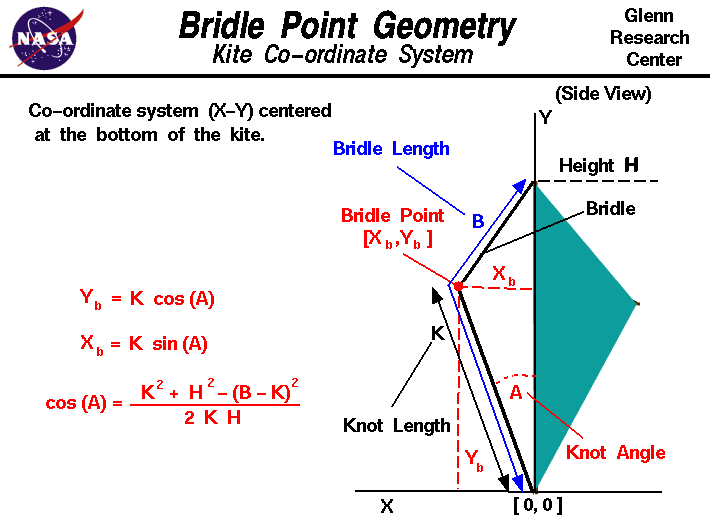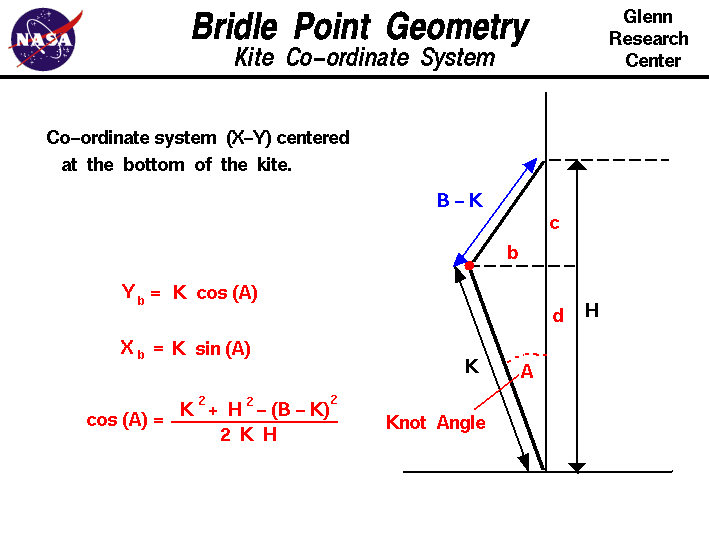+ Text Only Site
+ Non-Flash Version
+ Contact GlennAn excellent way to gain an understanding and a feel for the interaction of forces on an object is to fly a kite. Kite flying is fun when done safely and you can study many of the fundamentals of airplane aerodynamics because a kite works very much like an airplane. There are, however, some important differences in the response of a kite to external forces that do not occur in an airplane. An airplane in flight rotates about its center of gravity which is the average location of the weight of all the parts of the airplane. A kite in flight does not rotate about its center of gravity because it is pinned by the bridle to the control line. In flight, the kite rotates about the bridle point which is the place where the line is attached to the bridle. (A kite in flight is more closely related to a hinged door than to an airplane in flight. The center of gravity of a hinged door is in the center of the door, but the door rotates about the hinges.) The location of the bridle point relative to the center of gravity (cg) and center of pressure (cp) determines the balance of torques on the kite and the trim angle at which the kite flies. On this page we show you how to compute the location of the bridle point. We have an installed an X-Y co-ordinate system on the kite with the origin at the bottom of the kite; the Y-axis is along the height H of the kite, the X-axis is perpendicular to the Y-axis and goes through the bottom of the kite. The X-axis is used as the reference line in the computation of cg and cp. The length of the bridle is called B and is measured from the origin to the top of the kite along the bridle string. The bridle is attached to the control line with a knot. The distance from the knot to the origin is length K. The knot (bridle point) is inclined at an angle to Y-axis which is called the knot angle A. The co-ordinates of the bridle point are Xb and Yb and from trigonometry: Yb = K * cos(A) Xb = K * sin(A) where cos and sin are the trigonometric cosine and sine functions. To determine the angle A, we need to do a little algebra. Here's a sketch of the bridle geometry with some auxiliary lines drawn in:We have split the triangle formed by the bridle into two right triangles. From the definition of cosine, we have: Eq. 1: cos(A) = d / K We need to find the value of d. From the construction we have: Eq. 2: c + d = H And from the Pythagorean Theorem applied to both triangles: Eq. 3: K^2 = d^2 + b^2 Eq. 4: (B - K)^2 = c^2 + b^2 Eliminate b^2 from these two equations by subtracting Eq. 4 from Eq. 3.: K^2 - (B - K)^2 = d^2 - c^2 Now substitute for c from Eq. 2: K^2 - (B - K)^2 = d^2 - (H - d)^2 K^2 - (B - K)^2 = d^2 - (H^2 - 2 * H * d + d^2) K^2 - (B - K)^2 = - H^2 + 2 * H * d Eq. 5 : [K^2 - (B - K)^2 + H^2] / (2 * H) = d Now substitute the value of d in Eq. 5 into Eq. 1: cos(A) = [K^2 + H^2 - (B - K)^2] / (2 * K * H) The KiteModeler computer program can be used to calculate the various geometric variables described on this page and their effects on kite performance. Activities: Guided Tours Navigation ..Beginner's Guide Home Page+ Inspector General Hotline + Equal Employment Opportunity Data Posted Pursuant to the No Fear Act + Budgets, Strategic Plans and Accountability Reports + Freedom of Information Act + The President's Management Agenda + NASA Privacy Statement, Disclaimer, and Accessibility CertificationEditor: Nancy Hall NASA Official: Nancy Hall Last Updated: May 13 2021 + Contact Glenn